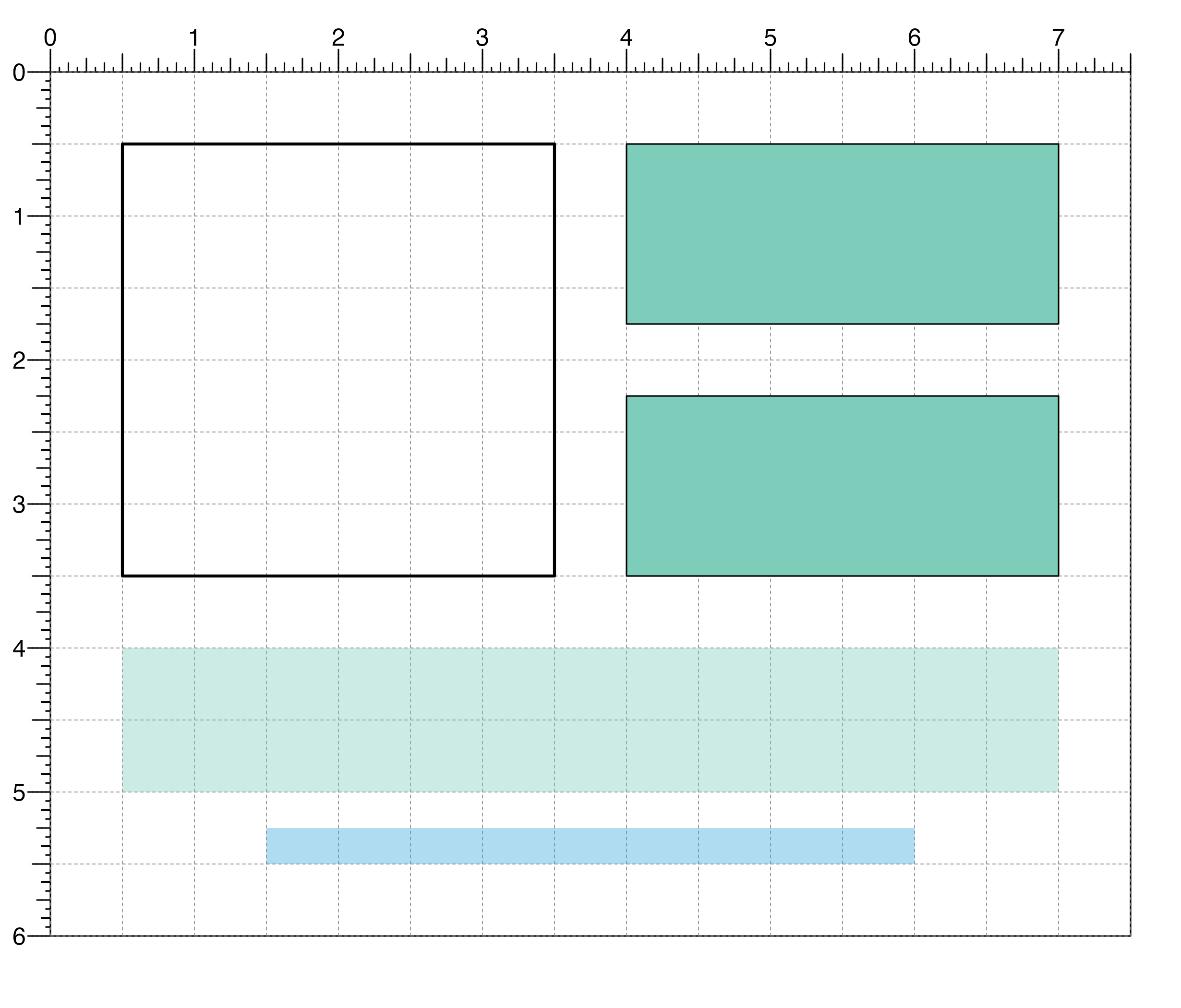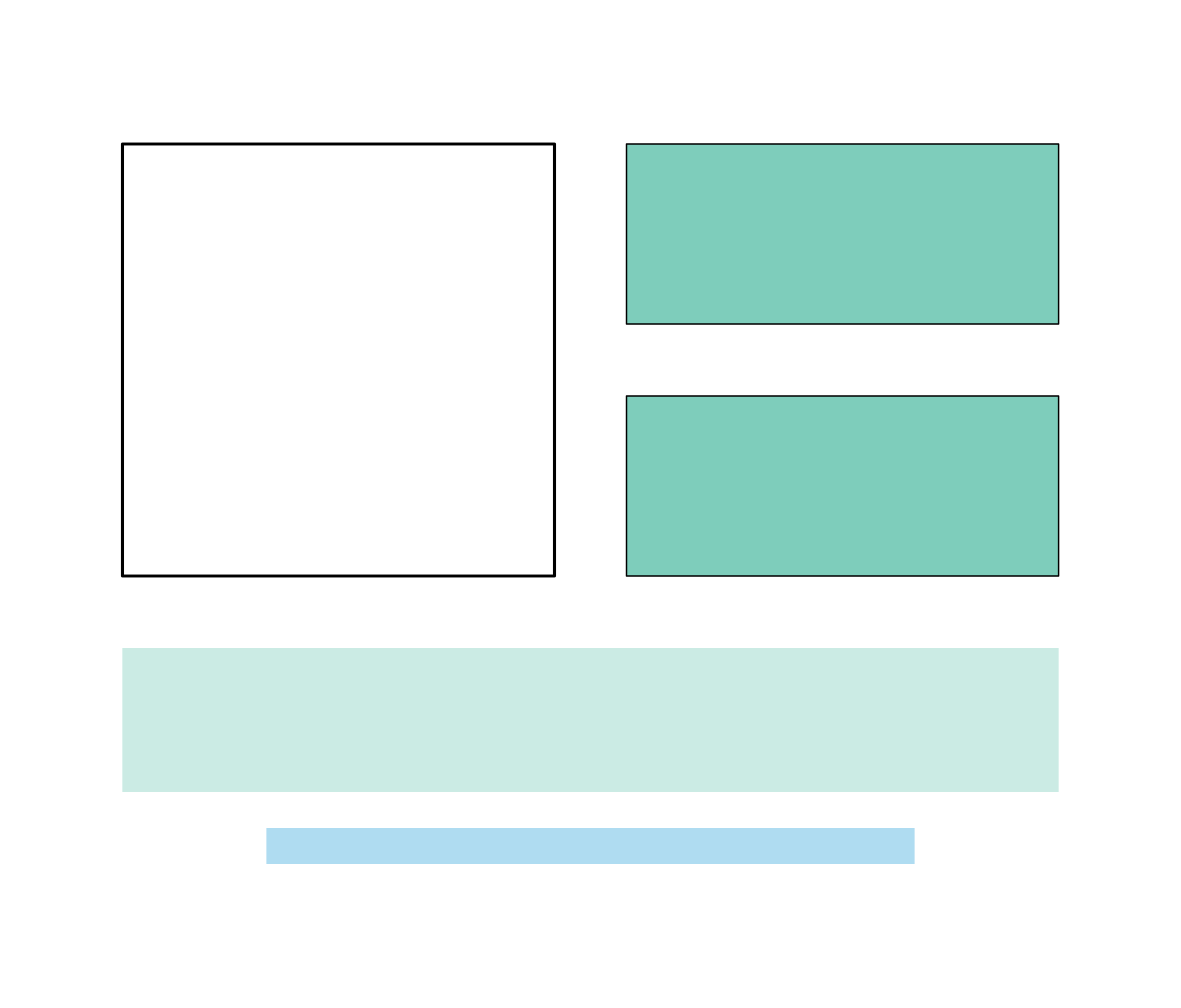Plot a rectangle within a plotgardener layout

plotRect(
x,
y,
width,
height,
just = "center",
default.units = "inches",
linecolor = "black",
lwd = 1,
lty = 1,
fill = NA,
alpha = 1,
params = NULL,
...
)

## Arguments

x

A numeric vector or unit object specifying rectangle x-locations.

y

A numeric vector, unit object, or a character vector of values containing a "b" combined with a numeric value specifying rectangle y-locations. The character vector will place rectangle y-locations relative to the bottom of the most recently plotted plot according to the units of the plotgardener page.

width

A numeric vector or unit object specifying rectangle widths.

height

A numeric vector or unit object specifying rectangle heights.

just

Justification of rectangle relative to its (x, y) location. If there are two values, the first value specifies horizontal justification and the second value specifies vertical justification. Possible string values are: "left", "right", "centre", "center", "bottom", and "top". Default value is just = "center".

default.units

A string indicating the default units to use if x, y, width, and height are only given as numerics or numeric vectors. Default value is default.units = "inches".

linecolor

A character value specifying rectangle line color. Default value is linecolor = "black".

lwd

A numeric specifying rectangle line width. Default value is lwd = 1.

lty

A numeric specifying rectangle line type. Default value is lty = 1.

fill

A character value specifying rectangle fill color. Default value is fill = NA.

alpha

Numeric value specifying color transparency. Default value is alpha = 1.

params

An optional pgParams object containing relevant function parameters.

...

Additional grid graphical parameters. See gpar.

## Value

Returns a rect object containing relevant placement and grob information.

## Examples

## Create a page
pageCreate(width = 7.5, height = 6, default.units = "inches")

## Plot one rectangle with no fill
plotRect(
x = 0.5, y = 0.5, width = 3, height = 3,
just = c("left", "top"), default.units = "inches",
lwd = 2, fill = NA
)
#> rect[rect1]

## Plot two rectangles with same width and height at different locations
plotRect(
x = 4, y = c(0.5, 2.25), width = 3, height = 1.25,
just = c("left", "top"), default.units = "inches",
fill = "#7ecdbb"
)
#> rect[rect2]

## Plot two rectangles with different widths, heights,
## locations, and colors
plotRect(
x = 3.75, y = c(4, 5.25), width = c(6.5, 4.5),
height = c(1, 0.25),
just = "top", default.units = "inches",
fill = c("#7ecdbb", "#37a7db"), linecolor = NA, alpha = 0.4
)
#> rect[rect2]## Hide page guides
pageGuideHide()